Evan Bremer

Assessing the Presence, Stability, and Linearity of Dominance Hierarchies Among Female Cichlids of the Species Astatotilapia burtoni

The African cichlid fish Astatotilapia burtoni has often been used in studies of social behavior; the dominance systems of the males are particularly well understood.  Studies focusing on the females of the species have been of their affiliative preference with respect to their reproductive status.  Since they school in the wild and in laboratory settings, the potential for the development of a social structure is significant.  I chose to study female A. burtoni with respect to their dominance structures and establish the groundwork for future studies of female dominance.  To do this, I observed two groups of female A. burtoni for a total of 8 weeks, recording their agonistic interactions.

I compared two recognized methods of calculating dominance hierarchies to calculate ranks for the fish each week, and assessed these rankings for linearity and stability.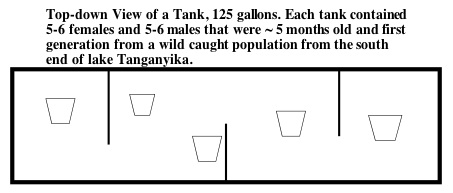The dominance score is calculated by dividing the number of victories by the number of agonistic interactions.

The dominance matrix is calculated by assigning each fish a score relative to each other fish and plotting these scores in a matrix.  A score of +1 was assigned to the fish in each dyad that has initiated more aggressive actions against the other, and the other fish in the dyad was assigned a score of -1.  If the fish tied, then both are assigned a score of 0.  The fish were then ranked by the sum of these scores from greatest to least.  Once these ranks have been established, greater weight is given to the outcomes of individual dyads.

The ranks alone do not fully describe a dominance hierarchy.

The linearity was measured with Landau's linearity index (h), which quantifies the probability that a given hierarchy can be explained without the inclusion of circular triads.  It is calculated with the following formula: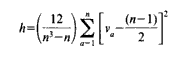where n = number of individuals, a = an individual and Va = number of individuals that individual outranks.

The stability over consecutive weeks was calculated by applying a Spearman rank correlation test.  This measure tells one how similar two sets of data are, and is only useful when comparing two sets of data.  It is calculated with the following formula: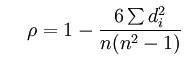where n = the number of pairs of values and di = the difference between the ranks in a pair.

My findings suggest that female A. burtoni form dominance hierarchies among themselves that are largely linear, but not stable over long durations.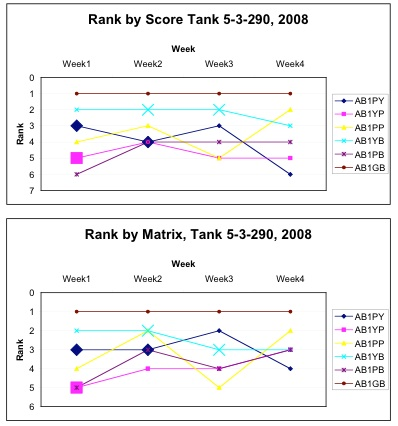These charts show the relatvie rank for each female in a single tank across the four weeks of observation. The dominance matrix method (a) and the dominance score method produce similar heirarchies.  (codes represent colored beads of female tag (P= pink, Y= yellow, B= blue, G= green). Larger symbols indicate brooding.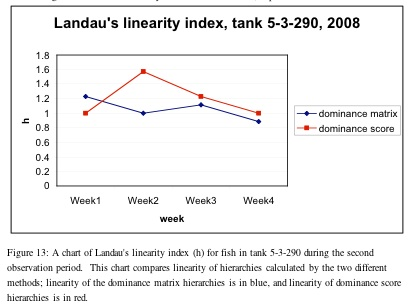The dominance score assumes a linear hierarchy, so Landau's linearity index values for hierarchies calculated by this method may be artificially high.  Since the dominance matrix and dominance score differ in their assumptions regarding linearity, a quantitative comparison is inappropriate.  Despite the above, it is possible to make a qualitative judgement on which method is better based on analyses of linearity.  Since circular triads were observed, the dominance score and other methods that assume linearity are probably wrong choices.  The dominance matrix is the best method analyzed here.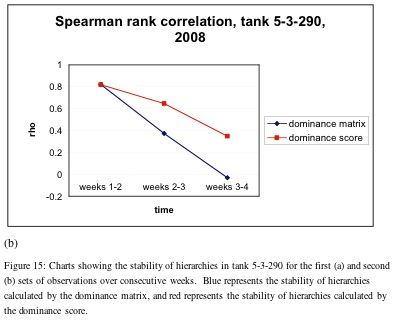Overall, stability over consecutive weeks is low (mean Spearman's rank correlation score: 0.27639 for dominance matrix and 0.39459 for dominance score.  Correlations between ranking methods on the other hand is high.

This kind of detailed analysis of A. burtoni females and their dominance structures has never been done before.  All the data needs to be verified, but I can conclude from this data that female A. burtoni do form dominance structures that are stable for at least 24 hours.  These structures are mostly linear, but circular triads occasionally arise.  It is possible that reproductive status has an effect on dominance, but this requires further study, and a longer observation period.